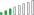cancel
Showing results for
Did you mean:Helper II

## multiply columns by one column, problem with data type changing

Hi All

I am transforming a bunch of columns by multiplying them on row level by values in a column:

Ex in tabel below I multiply Column2, Column3 & Column4 by Column1 (all columns 1-4 are data type: decimal numbers)

 Column1 Column2 Column3 Column4 2 1 2 1 3 1 1 3

Using:

Table.FromRecords(Table.TransformRows(#"Changed Type", (x) => Record.TransformFields(x, List.Transform({"Column2", "Column3", "Column4"}, (y) => {y, each x[Column1] * _}))))

And get:

 Column1 Column2 Column3 Column4 2 2 4 2 3 3 3 9

Wierd thing is that pre transformation Column 2, 3 & 4 are Decimal Numbers and post transformation they are datat type: any so I transforma all the columns to decimal number in next step.
And I´m finding some smal errors that feels like rounding errors on some rows.

Anyone out there with an Idea what I´m doing wrong?

1 ACCEPTED SOLUTIONCommunity Support

Hi @MansRydell ,

You could use the Table.TransformColumnTypes function to change all the data types to decimal number.

``````= let
output=Table.FromRecords(Table.TransformRows(#"Changed Type", (x) => Record.TransformFields(x, List.Transform({"Column2", "Column3", "Column4"}, (y) => {y, each x[Column1] * _}))))
in
Table.TransformColumnTypes(output,List.Transform(Table.ColumnNames(output), each {_, type number}))``````Table.TransformColumnTypes - PowerQuery M | Microsoft Learn

List.Transform - PowerQuery M | Microsoft Learn

Best Regards,

Stephen Tao

If this post helps, then please consider Accept it as the solution to help the other members find it more quickly.

4 REPLIES 4Resolver III

Hi,

Try this solution with Table.ReplaceValue

`letSource = Table.FromRows(Json.Document(Binary.Decompress(Binary.FromText("HYyxDQAgDMN+6ZyhNEDKLYj/36DqZCm2cq8JyTQYsTmKC6FZ3NhOe7hlOFYbnsKEnygOLKqDgKsGQVI/eXYXSnvvAw==", BinaryEncoding.Base64), Compression.Deflate)), let _t = ((type nullable text) meta [Serialized.Text = true]) in type table [Column1 = _t, Column2 = _t, Column3 = _t, Column4 = _t]),Number_Type = Table.TransformColumnTypes( Source, {{"Column1", type number}, {"Column2", type number}, {"Column3", type number}, {"Column4", type number}}),Replace_Value = Table.ReplaceValue( Number_Type, each [Column1], "", (x,y,z) => x*y, {"Column2", "Column3", "Column4"}),Restore_Type = Value.ReplaceType( Replace_Value, Value.Type( Number_Type ))inRestore_Type`

StéphaneHelper II

Thanks everyone for you´re input. Unfortunately my issue is unresolved thanks for you´re effort if I find a solution I´ll post it. 🙏Community Support

Hi @MansRydell ,

You could use the Table.TransformColumnTypes function to change all the data types to decimal number.

``````= let
output=Table.FromRecords(Table.TransformRows(#"Changed Type", (x) => Record.TransformFields(x, List.Transform({"Column2", "Column3", "Column4"}, (y) => {y, each x[Column1] * _}))))
in
Table.TransformColumnTypes(output,List.Transform(Table.ColumnNames(output), each {_, type number}))``````Table.TransformColumnTypes - PowerQuery M | Microsoft Learn

List.Transform - PowerQuery M | Microsoft Learn

Best Regards,

Stephen Tao

If this post helps, then please consider Accept it as the solution to help the other members find it more quickly.Solution Sage

Hi @MansRydell,

Alternatively, you could give something like this a go.

Copy this code into a new blank query

``````let
Source = Table.FromRows(Json.Document(Binary.Decompress(Binary.FromText("i45WMlLSUTIEYggdqxOtZAwVAWFjpdhYAA==", BinaryEncoding.Base64), Compression.Deflate)), let _t = ((type nullable text) meta [Serialized.Text = true]) in type table [Column1 = _t, Column2 = _t, Column3 = _t, Column4 = _t]),
ChType = Table.TransformColumnTypes(Source,{{"Column1", Int64.Type}, {"Column2", Int64.Type}, {"Column3", Int64.Type}, {"Column4", Int64.Type}}),
ReplaceValue = List.Accumulate(
List.Skip( Table.ColumnNames(Source), 1),
ChType,
(s, a) => Table.ReplaceValue( s,
each Record.Field(_, a),
each Record.Field(_, a) * Record.Field(_, "Column1"),
Replacer.ReplaceValue, {a}
)
),
RestoreType = Value.ReplaceType( ReplaceValue, Value.Type( ChType ))
in
RestoreType``````

There are a number of transformations that can't retain type, above type is restored as it was before.

Here's a link to a good post that deals with rounding in M